#### Vol. 37, No. 2, 1971

 Download this articleFor screen For printingRecent Issues Vol. 322: 1  2 Vol. 321: 1  2 Vol. 320: 1  2 Vol. 319: 1  2 Vol. 318: 1  2 Vol. 317: 1  2 Vol. 316: 1  2 Vol. 315: 1  2Online Archive Volume: Issue:The Journal Subscriptions Editorial Board Officers Contacts Submission Guidelines Submission Form Policies for Authors ISSN: 1945-5844 (e-only) ISSN: 0030-8730 (print) Special Issues Author Index To Appear Other MSP Journals
The asymptotic behavior of norms of powers of absolutely convergent Fourier series

### Dennis Michael Girard

Vol. 37 (1971), No. 2, 357–381
##### Abstract

Let f(t) have an absolutely convergent Fourier series f(t) = akeikt and set f= |ak|. In this paper we will study the asymptotic behavior of fnas n →∞. THEOREM. Let f be absolutely continuous and let fbe of bounded variation on the real line modulo 2π. Let f(0) = 1 but |f(t)| < 1 for t0 and suppose that for t near 0, f(t) = ϕ(eit) where ϕ is defined and analytic near z = 1. Define the parameters α,p,q, A and β as follows

 α = ϕ(1) ϕ(z) = zα + Aip(z − 1)p + o(1)(z − 1)p,z → 1(A≠0) |ϕ(eit)| = 1 − βtq + o(tq),t → 0(β≠0).
Then, (a) for pq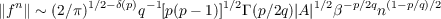where δ(p) = 0 if p is even and, = 1 if p is odd; (b) for p = q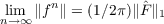where F is the Fourier transform of F(t) = exp(Atp). The following results about these parameters are known and easily verifiable: p and q are positive integers, 2 p q, q is even, β > 0,ReA 0;p = q if and only if ReA0;p = q implies β = ReA;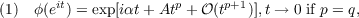and

 (2) ϕ(eit) = exp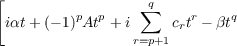+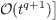, as t → 0,c r real, if p≠q.
We outline in §5 how it is possible to relax the condition of analyticity at t = 0 and replace it with conditions (1) and (2) where the 𝒪 terms satisfy certain smoothness conditions. G. W. Hedstrom proved in 1966 that under these same hypotheses, there exist two constants c,C such that c < fnn(1p∕q)2 < C

Primary: 42.12
##### Milestones